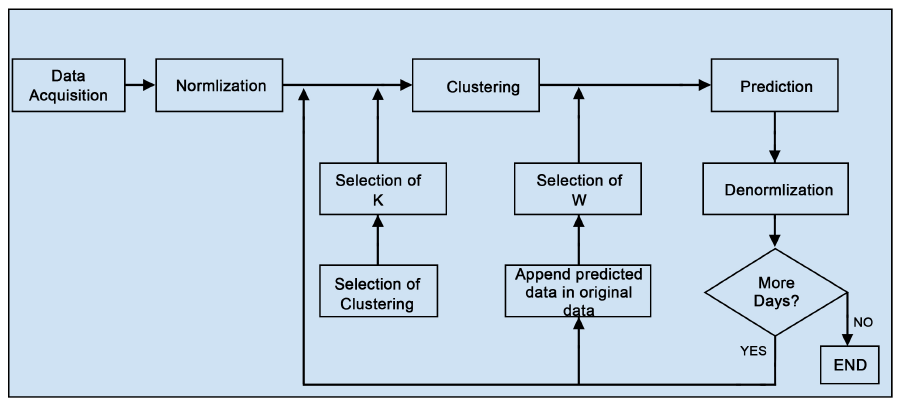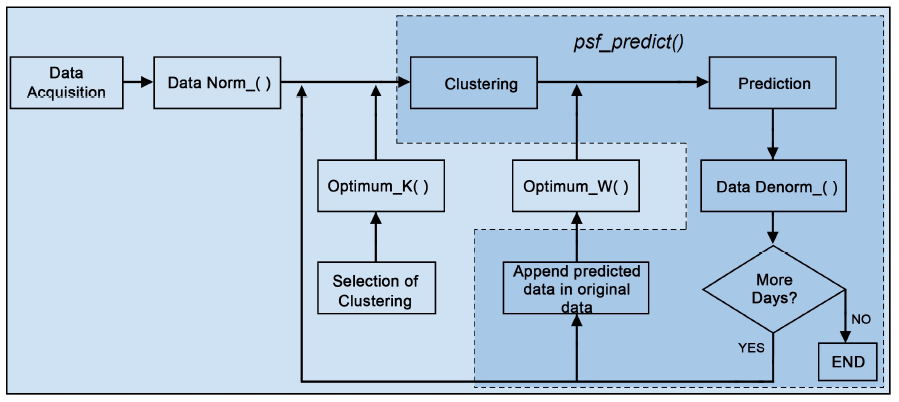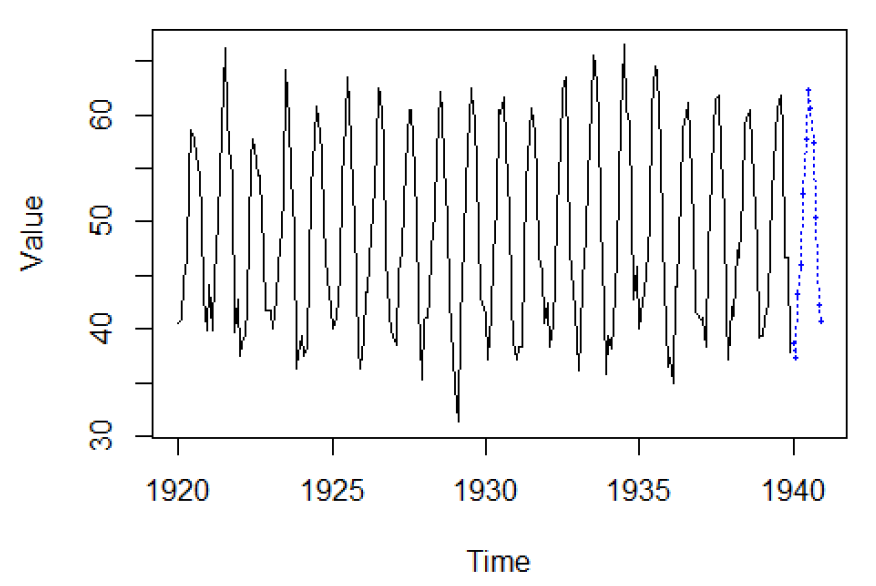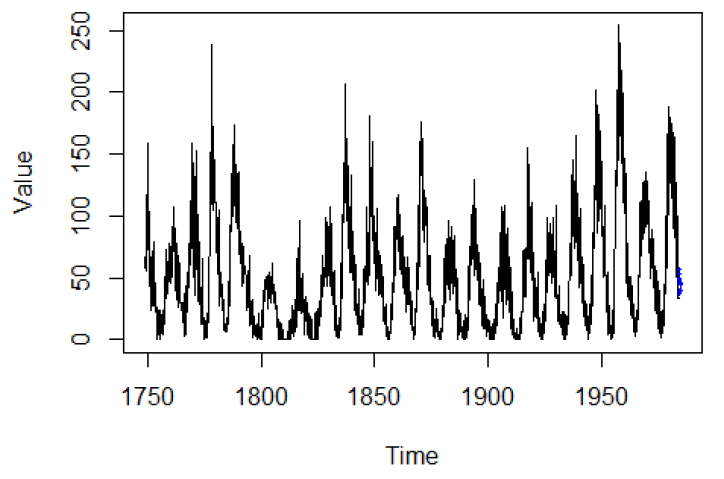# PSF: a good alternative to the ARIMA method for seasonal univariate time series forecasting

Challenges in univariate time series analysis:

Time series analysis plays an important role in numerous applications such as healthcare, economics, finance, environment, climate, agriculture, research, energy, and many more. There are several aspects of time series analysis, which includes predictions, univariate forecasts, missing value imputations, outlier detections, time series transformation, and cleaning, etc. In most of the applications, the final goal is to achieve an accurate prediction or forecasting results. Out of these, forecasting a univariate time series is one of the challenging tasks, since such a process needs to understand, recreate, and extrapolate the patterns available in the targeted time series itself. Whereas, in the multivariate prediction process, there can be the availability of several variables with which predicting process usually becomes easier. This is the reason that there are very few methodologies are available for univariate time series forecasting than the multivariate prediction ones.

The ‘Forecast’ package in R is a boon for time series analysis, which provides several features and benchmarks including ARIMA and ETS methods and corresponding user-friendly interface. In this blog, I am introducing and demonstrating a very interesting R package for time series forecasting, which can be an excellent alternative and replacement for the benchmarked forecasting methods, especially for the time series with seasonal characteristics.

Yes, it is the ‘Pattern Sequence-based forecasting algorithm’, popularly known as ‘PSF’. It is a successful forecasting method based on the assumption that there exist pattern sequences in the time series. It was proposed in the year 2011 and published here.

No worries, if you don’t have access to IEEE journals, there is another related open-source publication related to PSF. You can download it here. This paper is an introduction to the R package of the PSF algorithm, which also described the PSF methodology in detail.

In this blog, I am going to discuss the introduction to PSF, its demonstration, performance, limitations, new updates, modifications, and possible future aspects of the PSF algorithm.

Brief introduction of PSF:

The PSF algorithm consists of several tasks, which are broadly divided into two steps, i.e., clustering of data and the forecasting based on clustered data. The PSF is a closed-loop process, hence it forecasts values up to long-duration by appending earlier forecasted value to existing original time series data. However, it is worthy to note that PSF is particularly developed to forecast time series exhibiting some patterns in the historical data, such as weather, electricity load, or solar radiation. The application of PSF to time series without such kind of inherent patterns might lead to the generation of not, particularly competitive results.

Following is the block diagram of the PSF method.Block diagram of the PSF method.

The technical details of PSF are not discussed in this blog. It is discussed in detail in the R journal article, here. PSF consists of various tasks including the optimum cluster size, window size selection, pattern searching, and prediction processes. These processes are performed in the R package, PSF with different functions including psf(), predict.psf(), plot.psf(), optimum_k(), optimum_w(), psf_predict() and convert_datatype(). All functions in the PSF package version 0.4 onwards are made private except for psf(), predict.psf() and plot.psf() functions, so that users could not use it directly. However, if users need to change or modify clustering techniques or procedures in private functions, the code in R is available at GitHub (https://github.com/neerajdhanraj/PSF). The block diagram of PSF method is transformed into the blocks of the PSF package as shown in the following figure.Block diagram with PSF package functions replacement for PSF algorithm.

The clustering of data is one of the initial and important tasks in PSF. In the PSF package, a k-means clustering technique for generating data clusters according to the time series data properties. The limitation of the k-means clustering technique is that the adequate number of clusters must be provided by users. Hence, to avoid such situations, the proposed package contains a function optimum_k() which calculates the optimum value for the number of clusters, (k), according to the Silhouette index. This function generates the optimum cluster size as output.

Once data clustering is performed, the optimum window size needs to be determined. This is an important but tedious and time-consuming process if it is manually done. The selection of optimum window size is done through cross-validation, in which data is partitioned into two subsets. In the PSF package, the optimum window size selection is done with the function optimum_w(), which takes as input the time-series data, the previously estimated k value, set candidate w values to search in, and the cycle of the input time series. This function estimates the optimum value for the window size such that the error between predicted and actual values is minimum. Like the optimum_k() function, the optimum_w() function is also a private function, and users cannot directly access it.

The PSF package exposes three functions to the user. The first one, psf(), can build a PSF model from a univariate time series. Once the PSF model is trained and returned, the user can invoke the S3 method predict() over the ‘psf’ object specifying the desired number of forecasted values via the n.ahead parameter. Finally, the third function exposed is the S3 method plot(), which produces a plot including both actual and predicted values from a PSF model and a numeric vector of predictions.

Internally, the psf_predict() function is one of the private functions which takes input the dataset in data.table format, as well as another integer inputs including a number of clusters (k), window size (w), a horizon of prediction (n.ahead), and the cycle parameter, which discovers the cycle pattern followed by the dataset. The psf() function uses optimum_k() and optimum_w() functions. The latter, in turn, uses the described psf_predict(). The function psf() returns the PSF trained model (S3 object of class ‘psf’) whose contents are described later in this section. The syntax for psf() function is shown below:

Within the indicated syntax, the parameter data is a univariate time series in any format, e.g. time series, vector, matrix, list, or data frame. Also, data must be strictly provided in numeric format. The parameter k is the number of clusters, whereas parameter w is the window size. Finally, the cycle parameter is the number of values that confirms a cycle in the time series. Usual values for the cycle parameter can be 24 hours per day, 12 months per year, or so on and it is used only when input data is not in the time-series format. If input data is given in time series format, the cycle is automatically determined by its internal frequency attribute. However, if the user provides a single value for either k or w, then this value is used in the PSF algorithm and the search for their optimum is skipped.

The plot.psf() function allows users to plot both the actual values of a series and predicted values obtained by a PSF model. This function takes the trained PSF model, denoted by the first parameter named x, which includes internally the original time series, obtained by psf(), along with the predicted values obtained through predict.psf(), and optionally other plot describing variables, like plot title, legends, etc.

or, simply

Demonstration of PSF package with an example:

The PSF package is a very handy tool for forecasting. The Forecast package is very popular for ARIMA and other methods due to its user-friendly functions such as ‘auto.arima( )’. On a similar ground, the PSF package is handy and the user need not to worry about the modeling. By default, the PSF package is fully automated, but it also allows users to operate manually as discussed earlier. Let’s see a sample example on standard time series to compare the performance of PSF package with ‘auto.arima( )’ and ‘ets( )’ functions from Forecast package, which are well-accepted functions in the R community working overtime series forecasting techniques.

In this example, standard time-series ‘nottem’ and ‘sunspots’ are used, which is available in R. The nottem dataset is the average air temperatures at Nottingham Castle in degrees Fahrenheit, collected for 20 years, on monthly basis. Similarly, sunspots dataset is mean relative sunspot numbers from 1749 to 1983, measured every month. For both datasets, all the recorded values except for the final year are considered as training data, and the last year is used for testing purposes.

In the following examples, model training, forecasting, and plotting were shown for the dataset nottem, but the procedure is the same for the dataset sunspots. In the first place, the model must be trained using the PSF package as is shown below.

Once the model is trained, forecasted values for the time series can be obtained using the S3 method predict() function, as is shown below.

To represent the prediction performance in plot format, the S3 method plot() can be used, as shown in the following code.forecasted results with function plot.psf() for nottem dataset.forecasted results with function plot.psf() for sunspots dataset.

The above plots show the forecasted results for nottem and sunspots time series. The forecasted values in both plots seem to follow the original patterns in the original time series. Further, the performance of PSF method is compared with ARIMA and ETS methods in terms of root mean square error (RMSE) values in the following tables.